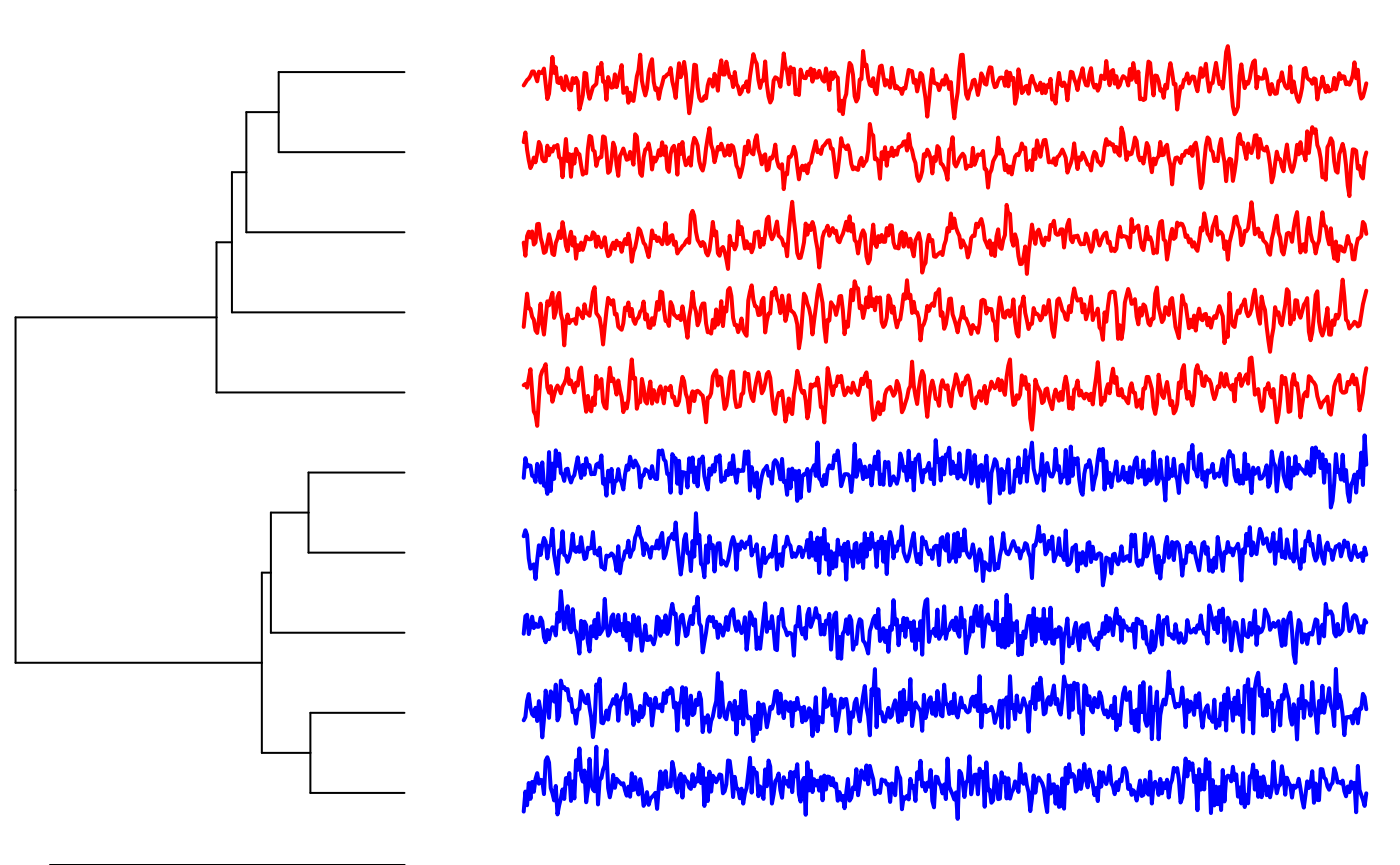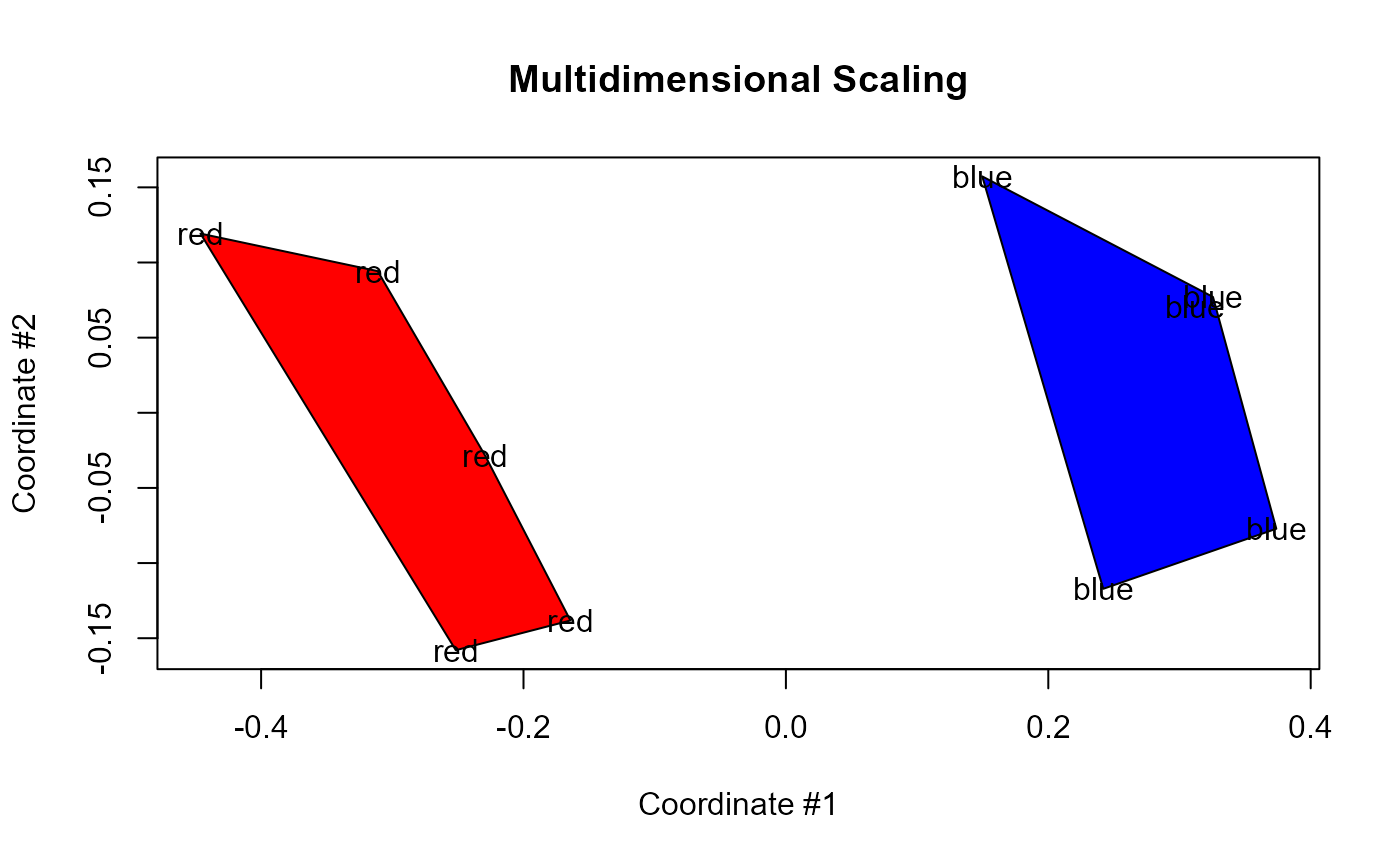library(pdc)

## Simulate data

# generate 5 ARMA time series for the first group
grp1 <- replicate(5, arima.sim(n = 500, list(ar = c(0.8897, -0.4858),
ma = c(-0.2279, 0.2488)),
sd = sqrt(0.1796)) )

# generate 5 ARMA time series for the second group
grp2 <- replicate(5, arima.sim(n = 500, list(ar = c(-0.71, 0.18),
ma = c(0.92, 0.14)),
sd = sqrt(0.291)) )

# combine groups into a single dataset
X <- cbind(grp1,grp2)

## Plot the data

plot(X[,1],type="l")plot(X[,6],type="l")# run clustering and color original groups each in red and blue
clustering <- pdclust(X)
colors <- c(rep("red",5),rep("blue",5))
plot(clustering, cols=colors)## MDS

mdsPlot(clustering,
labels = colors,
col = c("red","blue"))